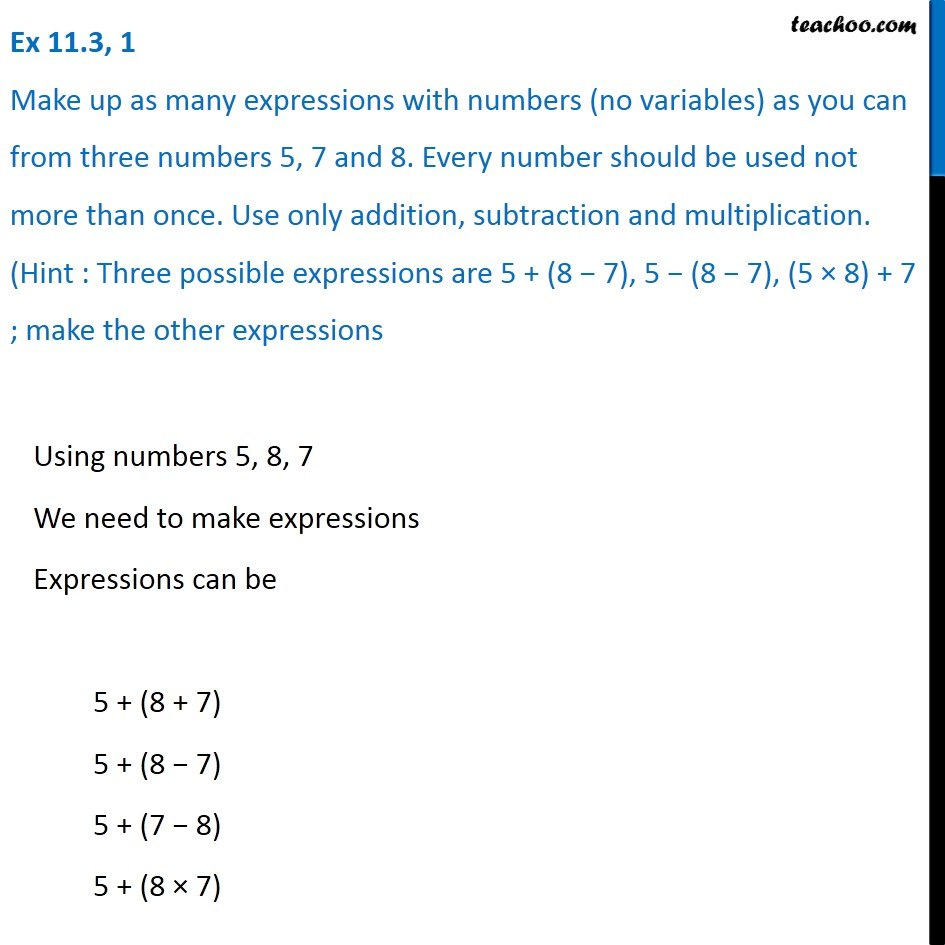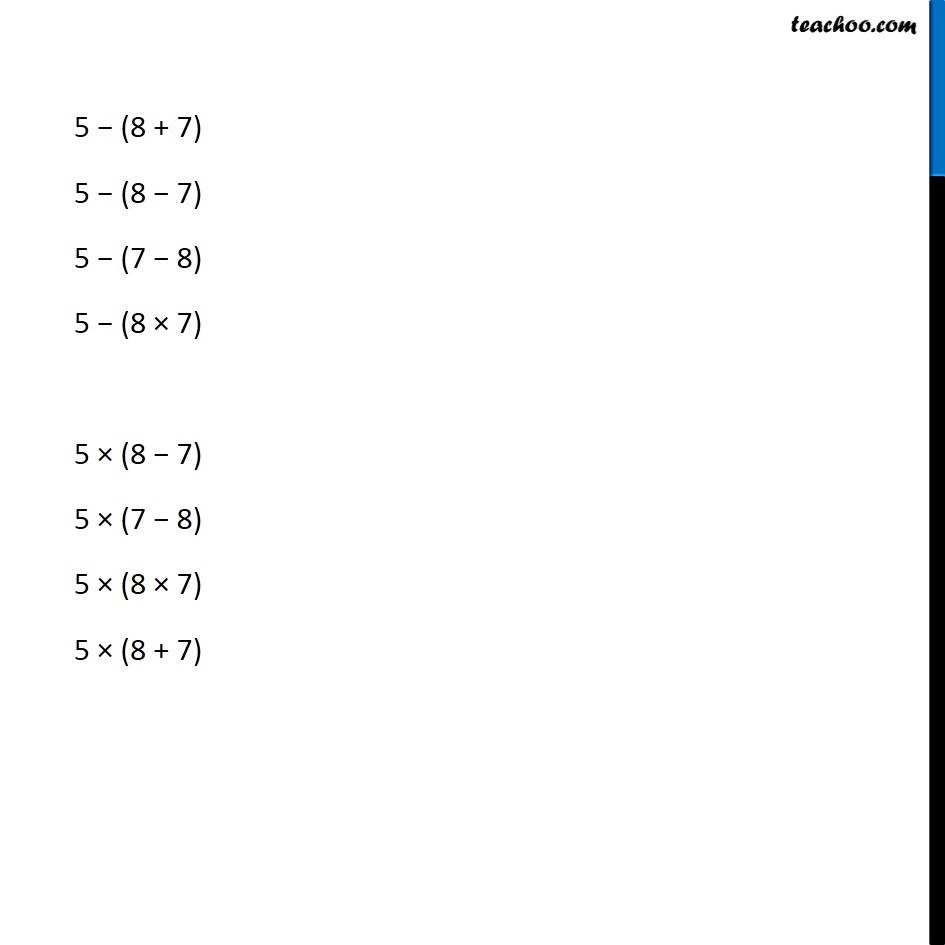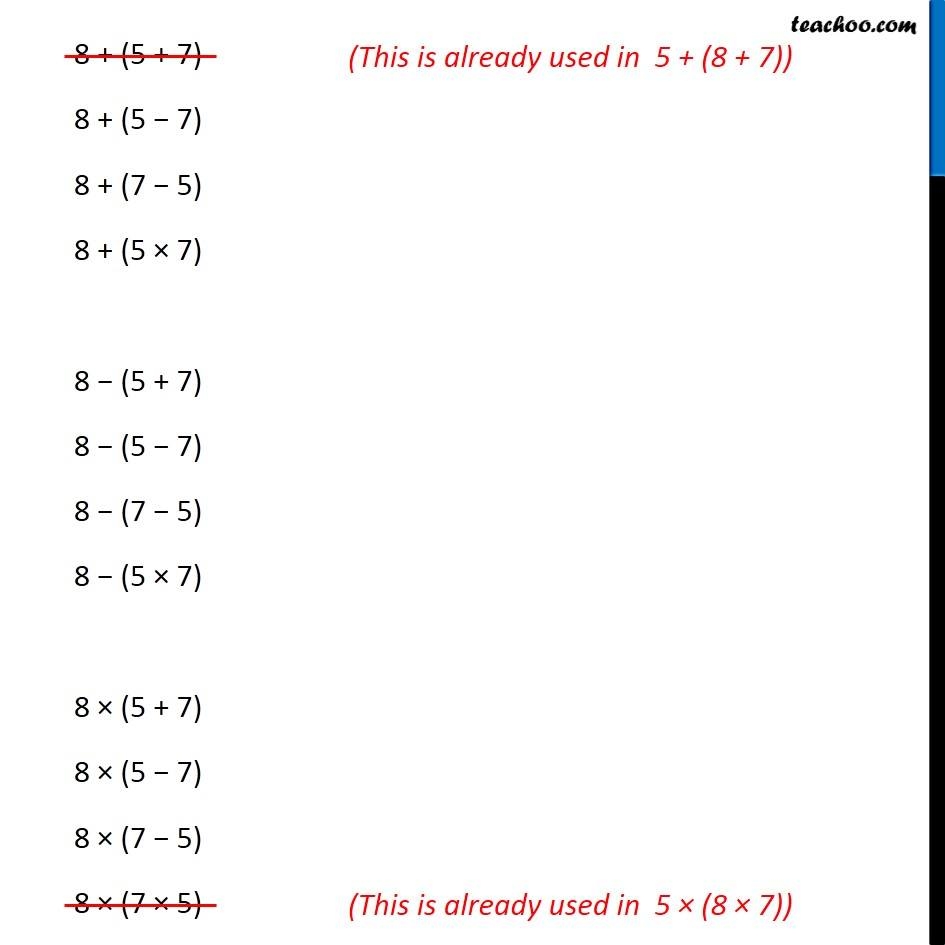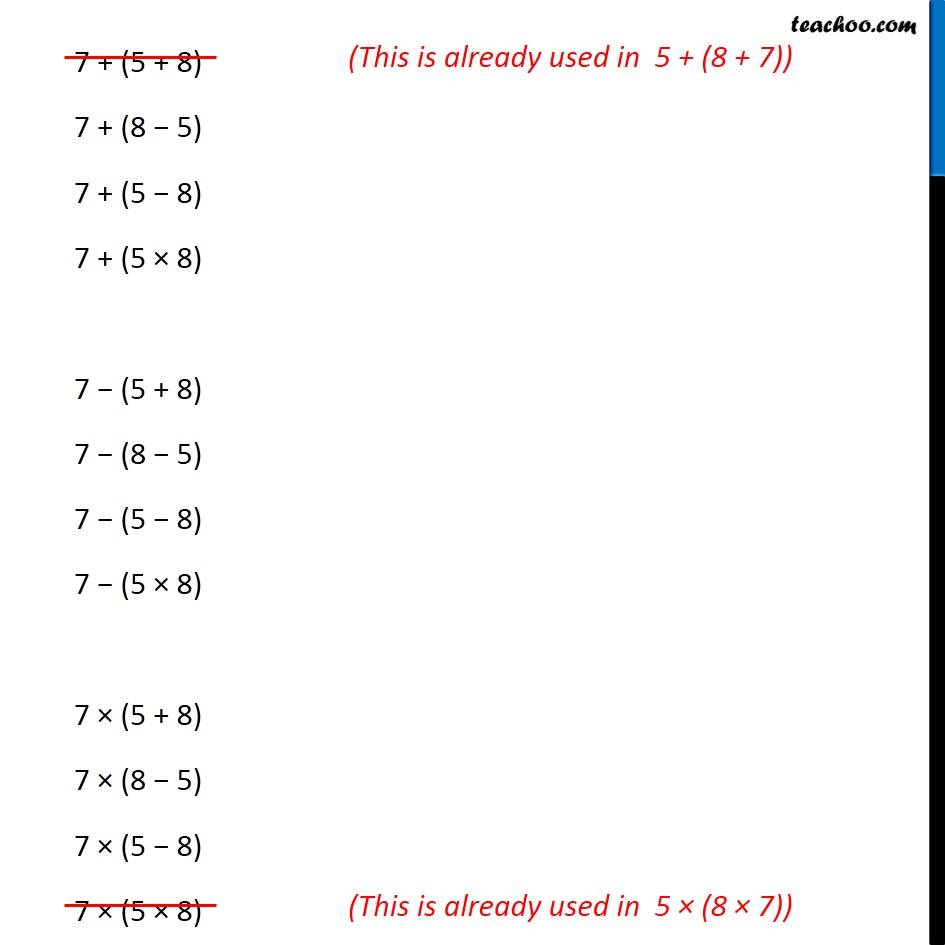Creating Basic Algebra Expressions - WorksheetLearn in your speed, with individual attention - Teachoo Maths 1-on-1 Class

### Transcript

Question 1 Make up as many expressions with numbers (no variables) as you can from three numbers 5, 7 and 8. Every number should be used not more than once. Use only addition, subtraction and multiplication. (Hint : Three possible expressions are 5 + (8 − 7), 5 − (8 − 7), (5 × 8) + 7 ; make the other expressions Using numbers 5, 8, 7 We need to make expressions Expressions can be 5 + (8 + 7) 5 + (8 − 7) 5 + (7 − 8) 5 + (8 × 7) 5 − (8 + 7) 5 − (8 − 7) 5 − (7 − 8) 5 − (8 × 7) 5 × (8 − 7) 5 × (7 − 8) 5 × (8 × 7) 5 × (8 + 7) 8 + (5 + 7) 8 + (5 − 7) 8 + (7 − 5) 8 + (5 × 7) 8 − (5 + 7) 8 − (5 − 7) 8 − (7 − 5) 8 − (5 × 7) 8 × (5 + 7) 8 × (5 − 7) 8 × (7 − 5) 8 × (7 × 5) 7 + (5 + 8) 7 + (8 − 5) 7 + (5 − 8) 7 + (5 × 8) 7 − (5 + 8) 7 − (8 − 5) 7 − (5 − 8) 7 − (5 × 8) 7 × (5 + 8) 7 × (8 − 5) 7 × (5 − 8) 7 × (5 × 8)